# Crossed Axes for Charts in PowerPoint 2013 for Windows

Learn how to add and alter the position of crossed axes in PowerPoint 2013 for Windows.

Author:

Product/Version: PowerPoint 2013 for Windows

OS: Microsoft Windows 7 and higher

In a PowerPoint column chart, Axes are typically positioned on the left and bottom of the Plot Area. However, there may be scenarios where your chart axes cross each other within the Plot Area rather than be located on its edges, as shown in Figure 1, below.Figure 1: Chart with crossed axes

Attaining such a crossed axes can be desirable due to a variety of reasons in both the Value and Category axes:

• For Value Axes: With a Value axis, you may see this behavior when you have both positive and negative values, as is the case with the data represented in Figure 1, above. Retaining your axis at the zero level may be desirable so that negative values show below the axis, and positive values are placed above. Fortunately, you do not have to do anything to cross the Value axis in such a scenario since crossing for a chart that has both positive and negative values is the default behavior. Look at the source data shown in Figure 2, note that it has both positive and negative values.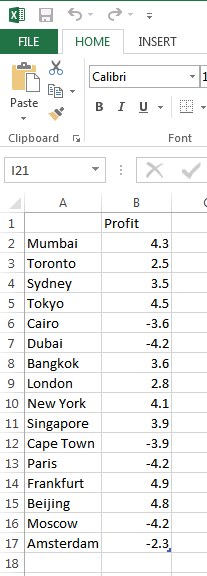Figure 2: Data with both positive and negative values

If the data shown in Figure 2, above is used to create a Column chart, then PowerPoint will automatically create a chart with a crossed Value axis at the zero value point, as shown in Figure 3.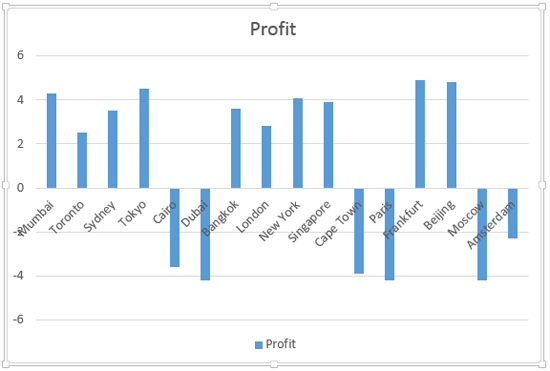Figure 3: Value axis is crossed at the zero value point

However, you are not limited to retaining the crossing position of the axis at zero. There's no reason why it cannot be -2 or +2, or whatever you want. Later in this tutorial, we will show you how you can alter the position where this axis is crossed.
• For Category Axes: On the other hand, the reason to go chose a crossed Category axis differs. You may want a crossed Category axis if you have too many categories, as already shown in Figure 3 above, where we have 16 categories. In such a scenario a left most placed axis may not help you ascertain column representation at the far right. Placing the axis in the center (refer to Figure 1, shown earlier on this page) may provide a better solution.

To learn how to add crossed axes in PowerPoint 2013 charts, or to learn how to change the position where they cross in, follow these steps:

1. Let us start with Category axis. Select the Category axis, and right-click to bring up the contextual menu you see in Figure 4. Choose the Format Axis option. If you do not get the Format Axis option in the contextual menu, you may have right-clicked on another chart element, make sure you then deselect anything in the chart, and then right-click on the Category Axis.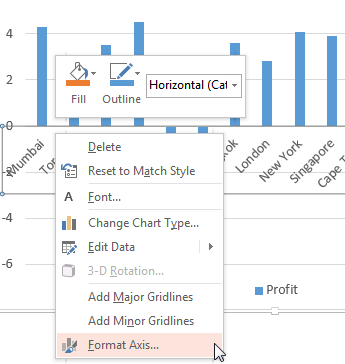Figure 4: Format Axis option selected
2. This opens the Format Axis Task Pane that you see in Figure 5. Make sure that the Axis Options button is clicked as shown highlighted in red within Figure 5. Then, select Axis Options (highlighted in blue within Figure 5). Note that the Vertical axis crosses option is set to Automatic, as shown highlighted in green within Figure 5.Figure 5: Default position where the Vertical axis crosses Horizontal axis
3. Select the At category number radio button as shown highlighted in red within Figure 6, and type the category number before which the axis should move. We typed 10 for our sample data (highlighted in blue within Figure 6).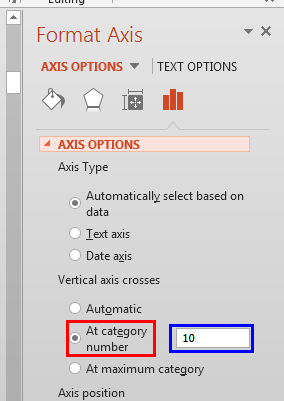Figure 6: Value axis crossing Category axis before 10th category

The resultant chart can be seen in Figure 7. This solution brings forth a problem of its own. The Axis Labels actually overlap one of the columns, as shown in Figure 7.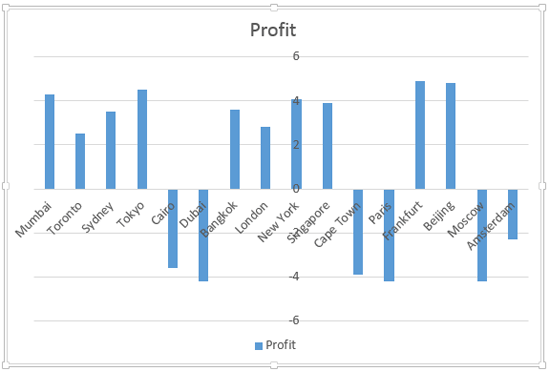Figure 7: The Value axes labels overlapping one of the columns
4. To solve this problem, select and right-click any chart element and within the resultant contextual menu, choose Edit Data option as shown in Figure 8, below. This opens a sub-menu, select Edit Data in Excel 2013 option (refer to Figure 8 again).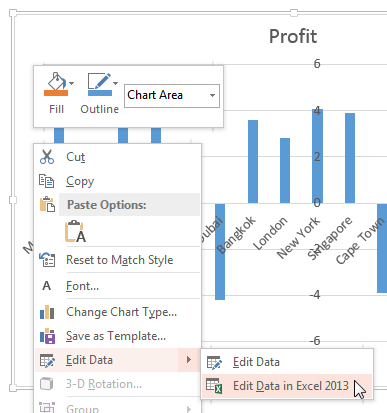Figure 8: Select Edit Data in Excel 2013 option
5. This brings up the same data that we last saw in Figure 2, earlier on this page. We will now insert a blank row after the 8th row, as shown in Figure 9.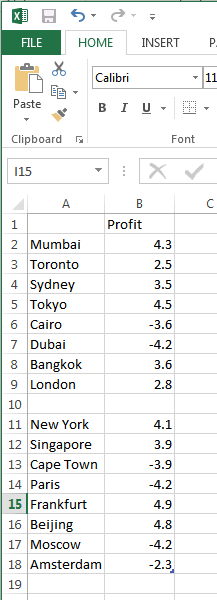Figure 9: Blank row added within the Excel data sheet
6. The resultant chart is so much clearer, notice the empty area that displays your Category axis labels so well in Figure 10, below. Do compare Figures 7 and 10 to see this difference!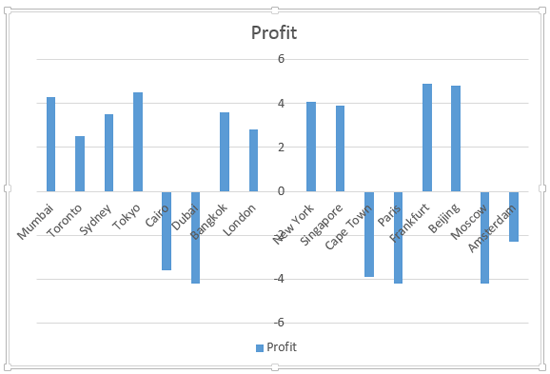Figure 10: A cleaner crossed axis
7. At this point you can optionally rotate the Category axis labels to make it sure that they don't overlap any of the Value axis labels. To do this, access the Format Axis Task Pane as explained in Step 1, earlier on this page. Within the Format Axis Task Pane, make sure that the Size & Properties button is selected as shown highlighted in red within Figure 11. Then, select the Alignment option (highlighted in blue within Figure 11). Thereafter, click the down-arrow (highlighted in green within Figure 11) provided with the Text direction option, from the resultant menu, choose the Rotate all text 90° option as shown in Figure 11.Figure 11: Select Rotate all text 90° option

In Figure 12 you can see the Category axis labels rotated to 90°.Figure 12: Category axis labels rotated to 90°
8. Now, let us consider Value axis. Notice that by default the Value axis is crossed by the Category Axis at the zero level (see Figure 12, above) with negative values below the axis, and positive ones above. Ideally, this is exactly how this should be. So, the only reason we are showing you how to change this crossed location is so that you can be aware of how to manually make this change. Select the Value axis, and right-click to bring up the same contextual menu you saw in Figure 4, earlier on this page. Choose the Format Axis option (refer to Figure 4 again).
9. This brings up the Format Axis dialog box as shown in Figure 13. Within the Format Axis Task Pane, Make sure that the Axis Options button is selected as shown highlighted in red within Figure 13. Then, select Axis Options (highlighted in blue within Figure 13). Thereafter, select the Axis value radio button (highlighted in green within Figure 13) and change the value to the point where you want the Value axis to be crossed by the Category Axis. We changed the value to -6 as shown highlighted in orange within Figure 13.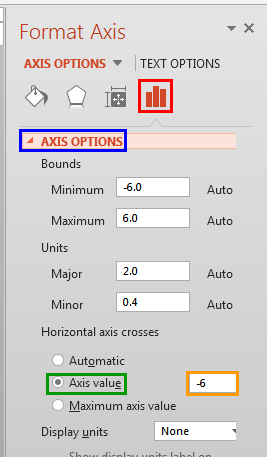Figure 13: Change the position where the Horizontal axis crosses the Vertical axis
10. This will move the Category axis down so that it crosses the Value axis at -6, as you can see in Figure 14.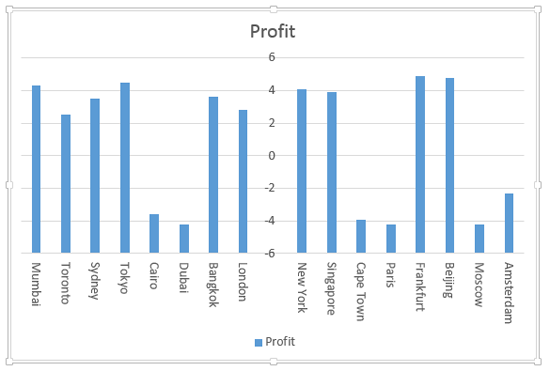Figure 14: Category axis crosses the Value axis at -6